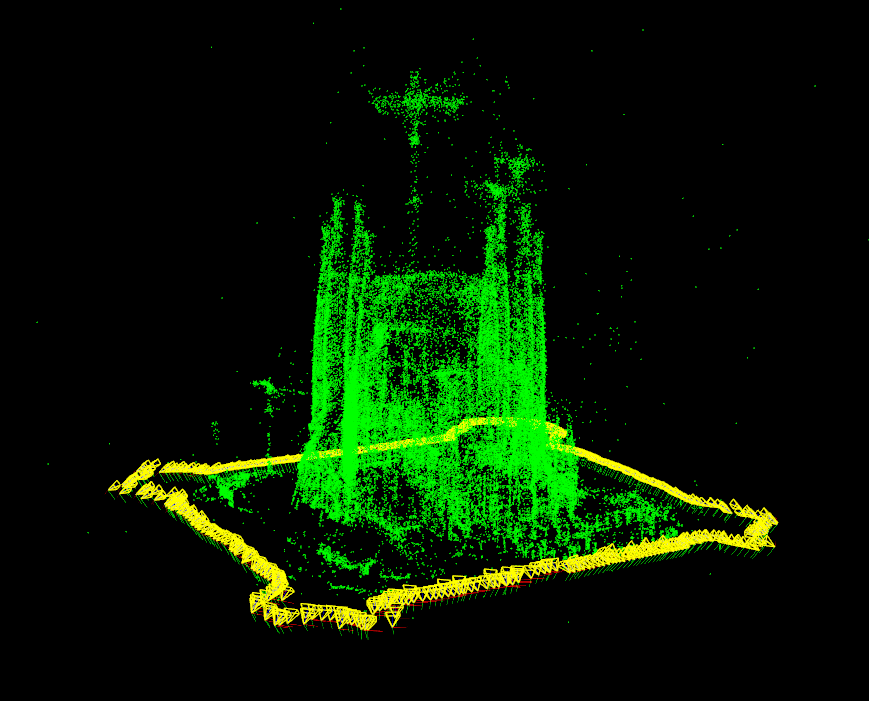OpenCV  3.4.0 Open Source Computer Vision
Import Reconstruction

## Goal

In this tutorial you will learn how to import a reconstruction from a given file obtained with Bundler :

• Load a file containing a set of cameras and 3D points.
• Show obtained results using Viz.

## Code

#include <opencv2/sfm.hpp>
#include <opencv2/viz.hpp>
#include <iostream>
using namespace std;
using namespace cv;
using namespace cv::sfm;
static void help() {
cout
<< "\n---------------------------------------------------------------------------\n"
<< " This program shows how to import a reconstructed scene in the \n"
<< " OpenCV Structure From Motion (SFM) module.\n"
<< " Usage:\n"
<< " example_sfm_import_reconstruction <path_to_file>\n"
<< " where: file_path is the absolute path file into your system which contains\n"
<< " the reconstructed scene. \n"
<< "---------------------------------------------------------------------------\n\n"
<< endl;
}
int main(int argc, char* argv[])
{
if ( argc != 2 ) {
help();
exit(0);
}
vector<Mat> Rs, Ts, Ks, points3d;
importReconstruction(argv, Rs, Ts, Ks, points3d, SFM_IO_BUNDLER);
viz::Viz3d window("Coordinate Frame");
window.setWindowSize(Size(500,500));
window.setWindowPosition(Point(150,150));
window.setBackgroundColor(); // black by default
vector<Vec3d> point_cloud;
for (int i = 0; i < points3d.size(); ++i){
point_cloud.push_back(Vec3f(points3d[i]));
}
vector<Affine3d> path;
for (size_t i = 0; i < Rs.size(); ++i)
path.push_back(Affine3d(Rs[i], Ts[i]));
viz::WCloud cloud_widget(point_cloud, viz::Color::green());
viz::WTrajectory trajectory(path, viz::WTrajectory::FRAMES, 0.5);
viz::WTrajectoryFrustums frustums(path, Vec2f(0.889484, 0.523599), 0.5,
viz::Color::yellow());
window.showWidget("point_cloud", cloud_widget);
window.showWidget("cameras", trajectory);
window.showWidget("frustums", frustums);
cout << endl << "Press 'q' to close each windows ... " << endl;
window.spin();
return 0;
}

## Results

The following picture shows a reconstruction from la Sagrada Familia (BCN) using dataset .Penate Sanchez, A. and Moreno-Noguer, F. and Andrade Cetto, J. and Fleuret, F. (2014). LETHA: Learning from High Quality Inputs for 3D Pose Estimation in Low Quality Images. Proceedings of the International Conference on 3D vision (3DV). URL